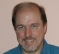## Real or complex FFT on IQ dataStarted by 7 years ago9 replieslatest reply 7 years ago9004 views

If I have a pair of quadrature signals I and Q which I want to perform FFTs on for spectral analysis. I am trying to understand what, if any, different information is present between performing two FFTs on I and Q separately or using a complex FFT (i.e. I + j*Q).

My understanding is that for the case of two FFTs on I and Q separately, the real (and mirrored) part of the spectrum will contain only information on the contribution from the cosine basis function for each frequency bin. For the case of the complex FFT, the real part of the spectrum is the same but the imaginary part of the spectrum represents the contribution from the sinusoid basis function.

Does this mean different (more?) information is present in the complex FFT as it includes information about the phase of the signal?

Thanks

[ - ]The information is the same.  Since the FFT is a linear operator, FFT(I + j*Q) = FFT(I) + j*FFT(Q).  Now break down each FFT as FFT(x) = DCT(x) - j*DST(x), and you can see where all the pieces are/go.

[ - ]In the end, you want to perform complex FFTs.  IQ data is basically modeling complex data, so, do a complex FFT.

[ - ]Thanks Tim, given the 90 degree phase shift I understand this reflects complex data but I am trying to get a grasp on how the information contained in the two approaches would differ.

[ - ]The way you stated it (the response to the cosine vs. the sine basis functions) is correct, but doesn't reflect what matters in the real world.  I can't think of a good way to state it generally, so I'll give you an example instead.

Say that you're doing IQ downconversion centered around 100MHz, with a 4MHz bandwidth.  If you have a signal at 101MHz, then doing the real FFT of each of the I and Q channels separately will show signals at plus and minus 1MHz.  With that same signal and the complex FFT, you'll see a signal at +1MHz only.

Basically the complex FFT will have complete and immediately sensible information.  The pair of real FFTs will have complete information, but you'd essentially have to use them to complete the complex FFT to get the "immediately sensible" part out of them.

[ - ]Thanks for the answer. I don't fully understand your comment "doing the real FFT of each of the I and Q channels separately will show signals at plus and minus 1MHz".

If I perform two real FFTs will the result not always be real and mirrored around DC?

I'm also confused around the cosine/sine basis function concept for the case of a real vs complex FFT. For a real input FFT we get a complex number out where atan(Re/Im) gives us the phase of the signal wrt a cosine basis function. For the complex input case what does the imaginary part of the spectrum represent - the phase wrt to a sine (90 degrees shifted)?

[ - ]I'm not sure what your mean by "real FFT" then -- an FFT can have real-valued inputs, but the output is going to be complex for all but some special-case inputs.

So what is a "real FFT" to you, then?

[ - ]Apologies for the confusion, The reason for mentioning phase was this is what I am ultimately interested in - I'm aware the FFT will have a complex output for either case.

What I was meaning was that a real FFT was the case for a purely real input and a complex FFT having a complex input signal.

[ - ]OK.  If you have an ordinary old FFT with a purely real (or, for that matter, purely imaginary) input, then the output will, by necessity, be mirrored around DC.  You know this, because you stated it.

So if I do a quadrature downconversion of a 101MHz signal with a 100MHz carrier, then both the inphase and quadrature parts will have 1MHz sine waves in them.  And if I treat each of these channels as real, and do FFTs on them, then the resulting FFTs will show tones at +1MHz and -1MHz.  Somehow, I think you know this -- or should know this -- too.